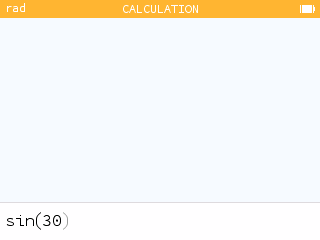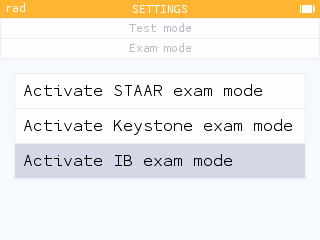## Grapher improvements

Editing an expression is now done directly in the cell that contains it.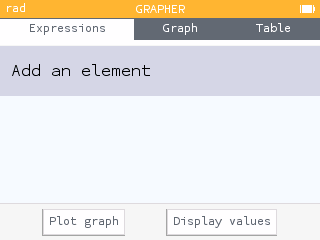The calculator is now able to plot a list of points in the form of `{(1,3),(2,3),(3,5)}`. It is also possible to plot a single point in the form of `(1,3)`.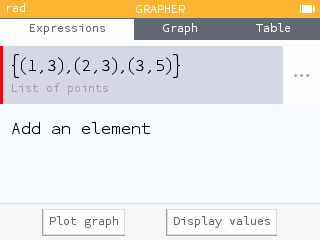You can now plot the scatterplot from the data in the Regression application by typing, for example `(X1,Y1)`.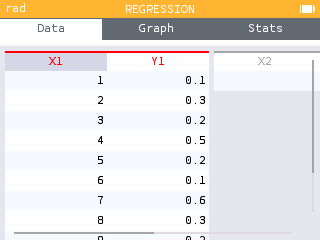Furthermore, the Grapher now plots the polar line whose equation is in the form `θ=a`.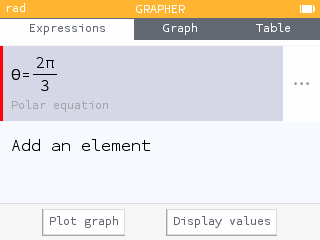## Equations

When a linear system has infinitely many solutions, the calculator now gives the parametrized form of the solutions.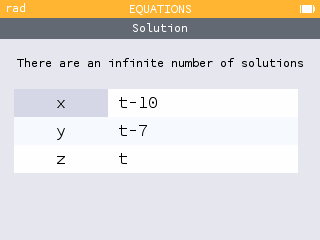## Distributions

In the Distributions application, it is now possible to calculate the value of μ or σ from a Normal probability distribution.

To do this, simply leave the value to be calculated (μ or σ) blank in the second step and then enter the value of the probability. The calculator will then give the requested value below the graph.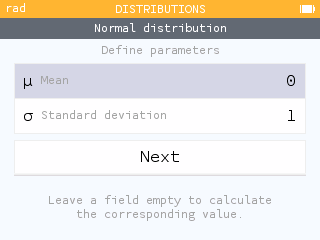## Regression and Statistics

The Regression application now automatically creates the function corresponding to the chosen model. You can find it in the Variables menu. This makes it possible to use the function in other applications such as the Grapher.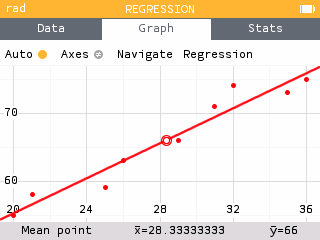The calculator now reports the standard deviation of residuals in the Stats tab of the Regression application.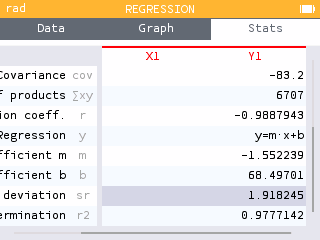The Data tabs now remembers the formula used when the user generated a column from a formula.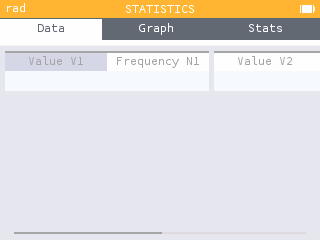You can now input a value when viewing the cumulative frequency plot and jump to the point on the graph.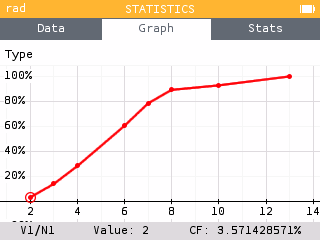## numpy module

It is now possible to use the `numpy` module of python. This module allows you to use arrays of 1 and 2 dimensions. You can now manipulate tables of numbers.

The functions available in the `numpy` module are listed in the Modules section of the toolbox key menu as well as in the Catalog.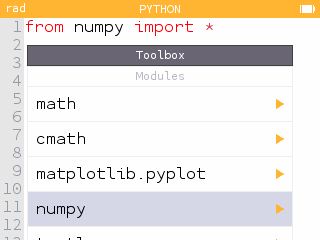In addition, the `gcd()` function has been added to the `math` module.

## But wait, there's more!

This version also offers many other improvements.

The Table tab of the Sequences application now gives the possibility to display a column of the sum of the terms of the sequence.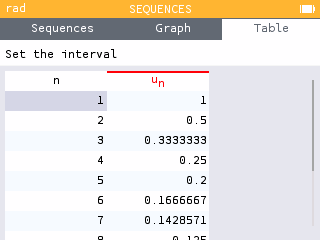Pressing the log key now displays the base number `10`. The base number can then be easily adjusted.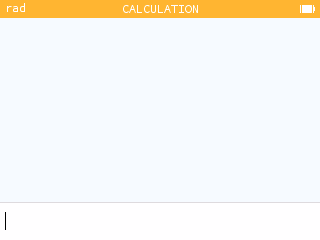When you use a trigonometric function in radian mode and the argument appears to be in degrees, the calculator reminds you that the angle unit is set to radians, and vice-versa.